# Math One Math Two Math Three Math Four

• Slides: 49Math One Math Two Math Three Math Four Math Five 100 100 100 200 200 200 300 300 300 400 400 400 500 500 500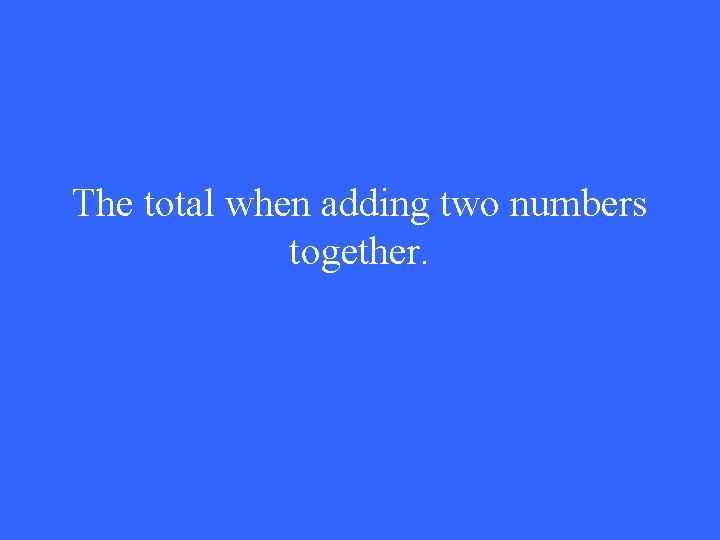The total when adding two numbers together.SumAltogether (+)An angle that measures less than 90 degrees.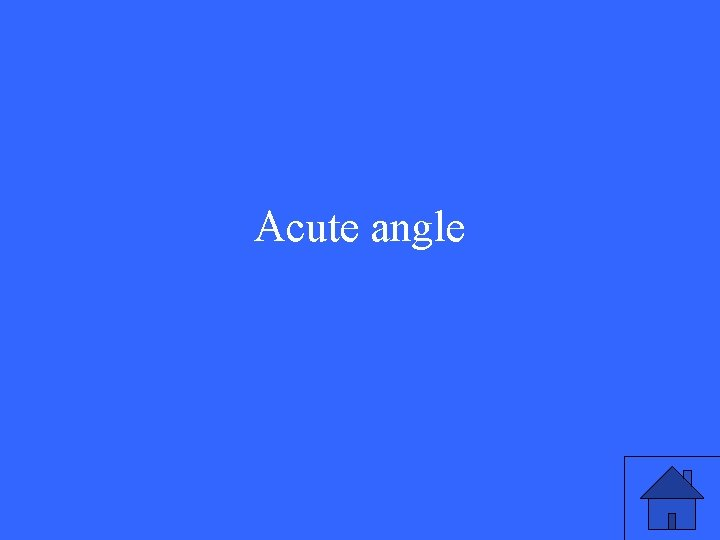Acute angleAn angle that measures between 90 and 180 degrees.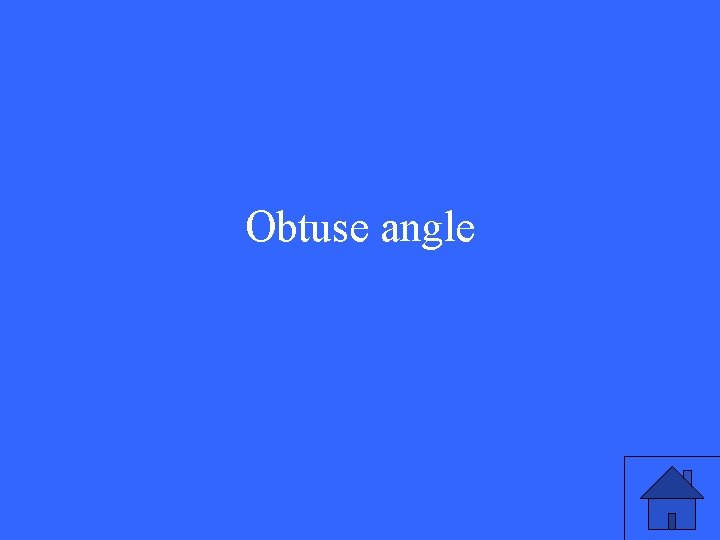Obtuse angleAn angle that measures exactly 90 degrees.Right AngleA line that goes left to right. The x-axis on a graphHorizontal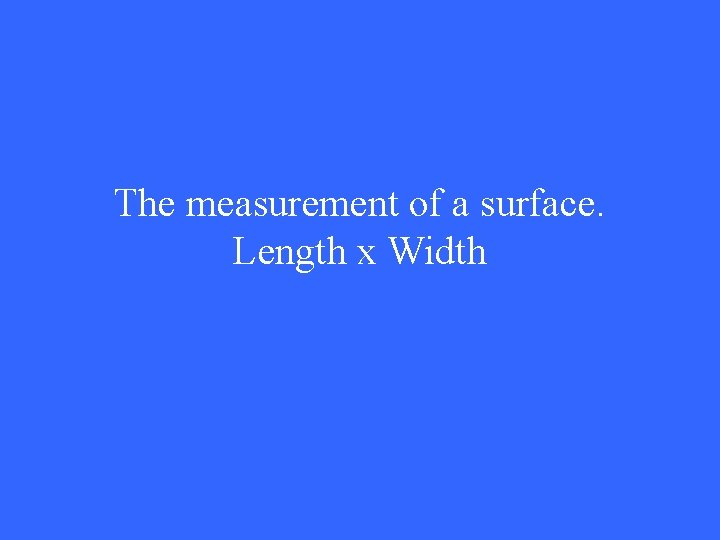The measurement of a surface. Length x Width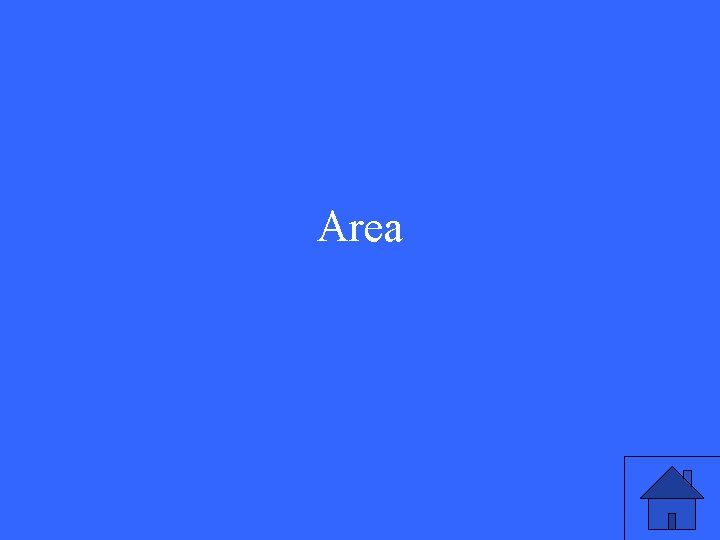Area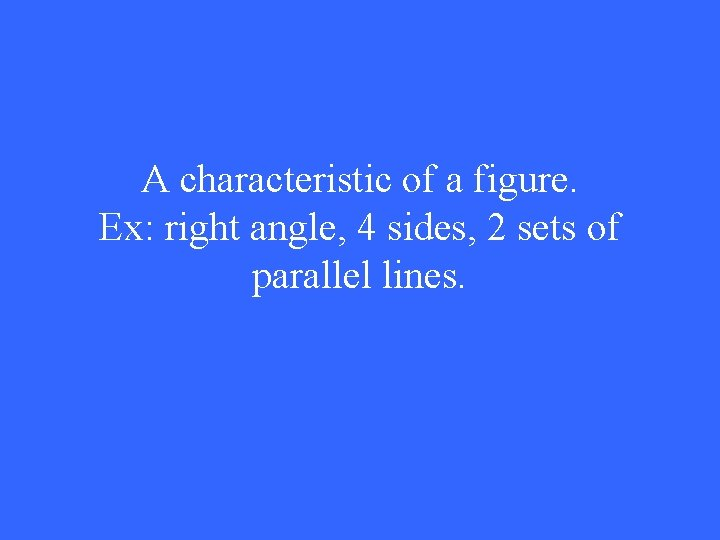A characteristic of a figure. Ex: right angle, 4 sides, 2 sets of parallel lines.AttributeA line that goes up and down. y-axis on a graphVertical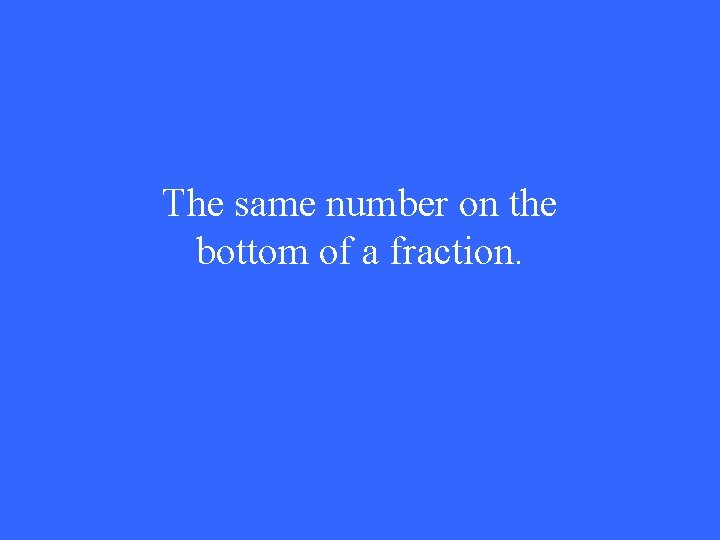The same number on the bottom of a fraction.Common DenominatorFind what is the same.CompareFinal decision or answerConclusion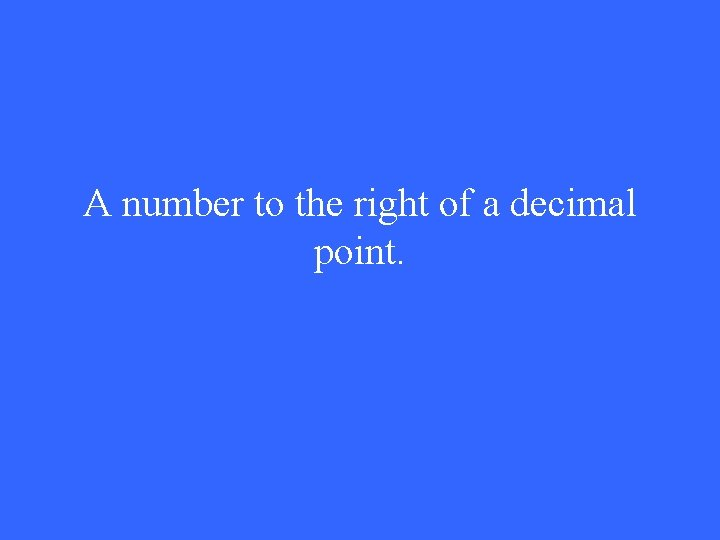A number to the right of a decimal point.Decimal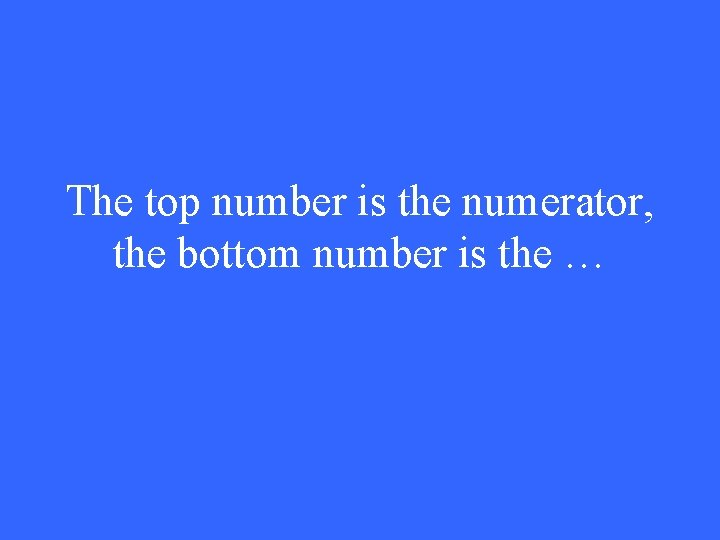The top number is the numerator, the bottom number is the …DenominatorA picture with labels.DiagramAlso known as subtraction (-)DifferenceA numberDigitThe lowest number.FewestPart of a whole.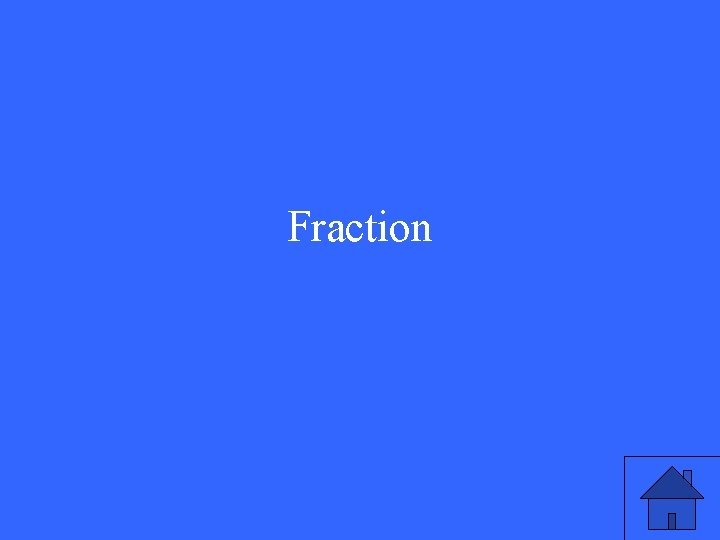FractionUnit of length equal to 1/12 of a foot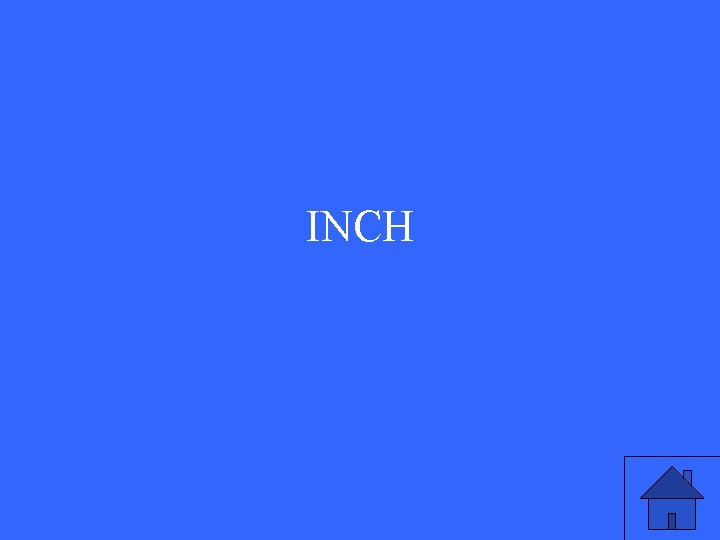INCHLargest or most value.GreatestA six sided polygonHexagonLarger numerator than denominator.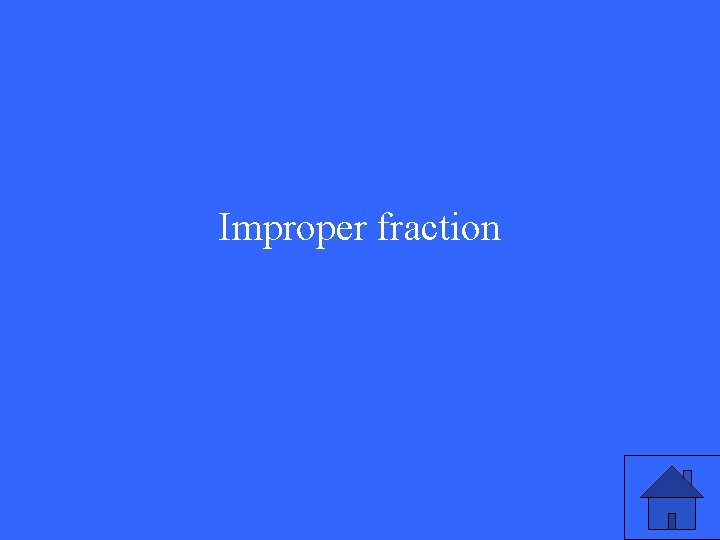Improper fractionProbably will happen.Most likely